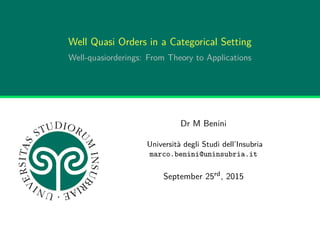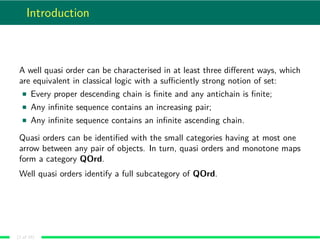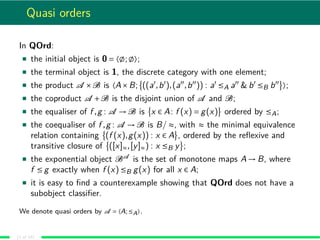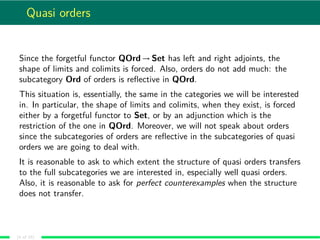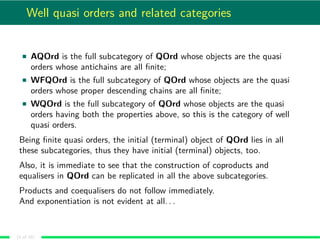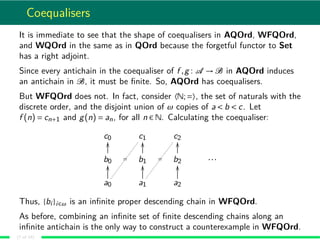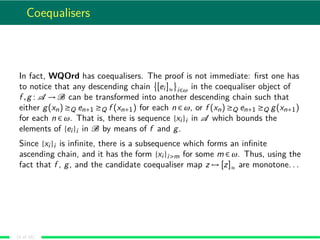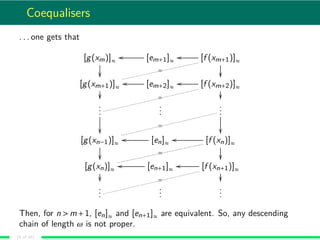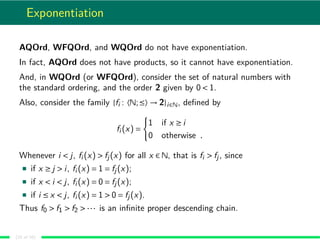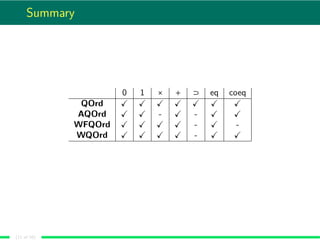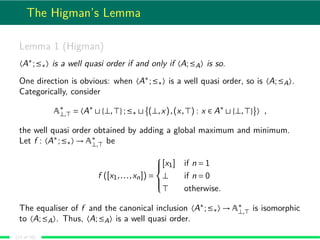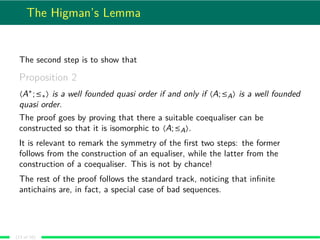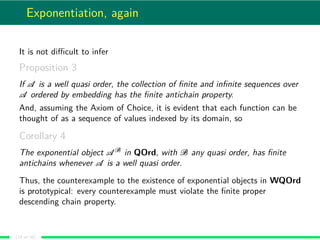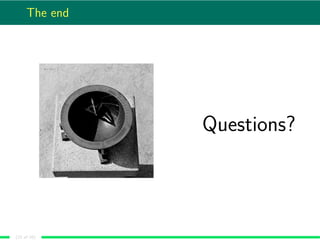1 of 15

### Well Quasi Orders in a Categorical Setting

1. Well Quasi Orders in a Categorical Setting Well-quasiorderings: From Theory to Applications Dr M Benini Università degli Studi dell’Insubria marco.benini@uninsubria.it September 25rd, 2015
2. Introduction A well quasi order can be characterised in at least three diﬀerent ways, which are equivalent in classical logic with a suﬃciently strong notion of set: Every proper descending chain is ﬁnite and any antichain is ﬁnite; Any inﬁnite sequence contains an increasing pair; Any inﬁnite sequence contains an inﬁnite ascending chain. Quasi orders can be identiﬁed with the small categories having at most one arrow between any pair of objects. In turn, quasi orders and monotone maps form a category QOrd. Well quasi orders identify a full subcategory of QOrd. (2 of 16)
3. Quasi orders In QOrd: the initial object is 0 = 〈 ; 〉; the terminal object is 1, the discrete category with one element; the product A ×B is 〈A×B; ((a ,b ),(a ,b )) : a ≤A a & b ≤B b 〉; the coproduct A +B is the disjoint union of A and B; the equaliser of f ,g : A → B is x ∈ A: f (x) = g(x) ordered by ≤A; the coequaliser of f ,g : A → B is B/ ≈, with ≈ the minimal equivalence relation containing (f (x),g(x)) : x ∈ A , ordered by the reﬂexive and transitive closure of ([x]≈,[y]≈) : x ≤B y ; the exponential object BA is the set of monotone maps A → B, where f ≤ g exactly when f (x) ≤B g(x) for all x ∈ A; it is easy to ﬁnd a counterexample showing that QOrd does not have a subobject classiﬁer. We denote quasi orders by A = 〈A;≤A〉. (3 of 16)
4. Quasi orders Since the forgetful functor QOrd → Set has left and right adjoints, the shape of limits and colimits is forced. Also, orders do not add much: the subcategory Ord of orders is reﬂective in QOrd. This situation is, essentially, the same in the categories we will be interested in. In particular, the shape of limits and colimits, when they exist, is forced either by a forgetful functor to Set, or by an adjunction which is the restriction of the one in QOrd. Moreover, we will not speak about orders since the subcategories of orders are reﬂective in the subcategories of quasi orders we are going to deal with. It is reasonable to ask to which extent the structure of quasi orders transfers to the full subcategories we are interested in, especially well quasi orders. Also, it is reasonable to ask for perfect counterexamples when the structure does not transfer. (4 of 16)
5. Well quasi orders and related categories AQOrd is the full subcategory of QOrd whose objects are the quasi orders whose antichains are all ﬁnite; WFQOrd is the full subcategory of QOrd whose objects are the quasi orders whose proper descending chains are all ﬁnite; WQOrd is the full subcategory of QOrd whose objects are the quasi orders having both the properties above, so this is the category of well quasi orders. Being ﬁnite quasi orders, the initial (terminal) object of QOrd lies in all these subcategories, thus they have initial (terminal) objects, too. Also, it is immediate to see that the construction of coproducts and equalisers in QOrd can be replicated in all the above subcategories. Products and coequalisers do not follow immediately. And exponentiation is not evident at all. . . (5 of 16)
6. Products Products in the subcategories of interest, when they exist, must have the same shape as in QOrd because it must be the right adjoint of the restriction of the diagonal functor. In WQOrd, ﬁnite products exist thanks to Dickson’s Lemma. The same holds in WFQOrd, by considering any proper descending chain in the candidate product, and constructing out of it two descending chains in the components, whose proper kernel must be ﬁnite, forcing the original chain to be ﬁnite too. But, AQOrd does not have all the binary products: consider 〈N;≤〉 and the (total) lexicographic order O on the free monoid over 0 < 1. Let xn = 0...01 be the word in O having n zeroes. Then {xn}n∈ω is an inﬁnite descending chain in O, so (n,xn) n∈ω deﬁnes an inﬁnite antichain in the product. This counterexample is perfect: pairing elements from an inﬁnite ascending chain with elements from an inﬁnite descending chain is the only way to construct a counterexample in AQOrd. (6 of 16)
7. Coequalisers It is immediate to see that the shape of coequalisers in AQOrd, WFQOrd, and WQOrd in the same as in QOrd because the forgetful functor to Set has a right adjoint. Since every antichain in the coequaliser of f ,g : A → B in AQOrd induces an antichain in B, it must be ﬁnite. So, AQOrd has coequalisers. But WFQOrd does not. In fact, consider 〈N;=〉, the set of naturals with the discrete order, and the disjoint union of ω copies of a < b < c. Let f (n) = cn+1 and g(n) = an, for all n ∈ N. Calculating the coequaliser: c0 c1 c2 b0 OO b1 OO b2 OO ··· a0 OO = a1 OO = a2 OO Thus, {bi }i∈ω is an inﬁnite proper descending chain in WFQOrd. As before, combining an inﬁnite set of ﬁnite descending chains along an inﬁnite antichain is the only way to construct a counterexample in WFQOrd. (7 of 16)
8. Coequalisers In fact, WQOrd has coequalisers. The proof is not immediate: ﬁrst one has to notice that any descending chain [ei ]≈ i∈ω in the coequaliser object of f ,g : A → B can be transformed into another descending chain such that either g(xn) ≥Q en+1 ≥Q f (xn+1) for each n ∈ ω, or f (xn) ≥Q en+1 ≥Q g(xn+1) for each n ∈ ω. That is, there is sequence {xi }i in A which bounds the elements of {ei }i in B by means of f and g. Since {xi }i is inﬁnite, there is a subsequence which forms an inﬁnite ascending chain, and it has the form {xi }i>m for some m ∈ ω. Thus, using the fact that f , g, and the candidate coequaliser map z → [z]≈ are monotone. . . (8 of 16)
9. Coequalisers . . . one gets that [g(xm)]≈ [em+1]≈ oo [f (xm+1)]≈ oo [g(xm+1)]≈ = [em+2]≈ oo [f (xm+2)]≈ oo = ... ... ... [g(xn−1)]≈ = [en]≈ oo [f (xn)]≈ oo = [g(xn)]≈ = [en+1]≈ oo [f (xn+1)]≈ oo = ... ... ... Then, for n m+1, [en]≈ and [en+1]≈ are equivalent. So, any descending chain of length ω is not proper. (9 of 16)
10. Exponentiation AQOrd, WFQOrd, and WQOrd do not have exponentiation. In fact, AQOrd does not have products, so it cannot have exponentiation. And, in WQOrd (or WFQOrd), consider the set of natural numbers with the standard ordering, and the order 2 given by 0 1. Also, consider the family {fi : 〈N;≤〉 → 2}i∈N, deﬁned by fi (x) = 1 if x ≥ i 0 otherwise . Whenever i j, fi (x) fj(x) for all x ∈ N, that is fi fj, since if x ≥ j i, fi (x) = 1 = fj(x); if x i j, fi (x) = 0 = fj(x); if i ≤ x j, fi (x) = 1 0 = fj(x). Thus f0 f1 f2 ··· is an inﬁnite proper descending chain. (10 of 16)
11. Summary 0 1 × + ⊃ eq coeq QOrd AQOrd - - WFQOrd - - WQOrd - (11 of 16)
12. The Higman’s Lemma Lemma 1 (Higman) 〈A∗ ;≤∗〉 is a well quasi order if and only if 〈A;≤A〉 is so. One direction is obvious: when 〈A∗ ;≤∗〉 is a well quasi order, so is 〈A;≤A〉. Categorically, consider A∗ ⊥, = 〈A∗ {⊥, };≤∗ (⊥,x),(x, ) : x ∈ A∗ {⊥, } 〉 , the well quasi order obtained by adding a global maximum and minimum. Let f : 〈A∗ ;≤∗〉 → A∗ ⊥, be f ([x1,...,xn]) =    [x1] if n = 1 ⊥ if n = 0 otherwise. The equaliser of f and the canonical inclusion 〈A∗ ;≤∗〉 → A∗ ⊥, is isomorphic to 〈A;≤A〉. Thus, 〈A;≤A〉 is a well quasi order. (12 of 16)
13. The Higman’s Lemma The second step is to show that Proposition 2 〈A∗ ;≤∗〉 is a well founded quasi order if and only if 〈A;≤A〉 is a well founded quasi order. The proof goes by proving that there a suitable coequaliser can be constructed so that it is isomorphic to 〈A;≤A〉. It is relevant to remark the symmetry of the ﬁrst two steps: the former follows from the construction of an equaliser, while the latter from the construction of a coequaliser. This is not by chance! The rest of the proof follows the standard track, noticing that inﬁnite antichains are, in fact, a special case of bad sequences. (13 of 16)
14. Exponentiation, again It is not diﬃcult to infer Proposition 3 If A is a well quasi order, the collection of ﬁnite and inﬁnite sequences over A ordered by embedding has the ﬁnite antichain property. And, assuming the Axiom of Choice, it is evident that each function can be thought of as a sequence of values indexed by its domain, so Corollary 4 The exponential object A B in QOrd, with B any quasi order, has ﬁnite antichains whenever A is a well quasi order. Thus, the counterexample to the existence of exponential objects in WQOrd is prototypical: every counterexample must violate the ﬁnite proper descending chain property. (14 of 16)
15. The end Questions? (15 of 16)